PHY294H - Lecture 18

Recommended problems for Lecture 18: FGT 29.8, 29.10, 29.11, 29.20
Recommended reading for Lecture 19: FGT pp 779-790

Introduction

There are many analogies between electrostatics and magnetostatics (time independent magnetic fields). Just as there are two types of electric charge, there are two types of magnetic charge''. They are called the North pole N'' and the south pole S''. Magnetic field lines come out of north poles and go into south poles. Two like poles repel and unlike poles attract. However there are no elementary particles which carry an isolated magnetic charge. That is, there is no particle that has magnetic charge N''. Instead elementary particles often have a property called spin which is quantized (for example the electron has spin half). Associated with spin is a quantum of magnetic dipole moment, not a quantum of magnetic charge. No free magnetic charges or magnetic monopoles'' have been observed, though there has been a lot of theoretical(Dirac) and experimental research looking for them. Magnetic dipoles occur as a property of elementary particles, for example a free electron which has spin 1/2 has magnetic dipole momentwhich is the Bohr magneton. The magnetic field lines due to a magnetic dipole (e.g.a bar magnet or an electron spin) look the same as the electric field lines due to an electric dipole.

However there is another way to produce magnetic fields. It was observed by Oersted and Ampere in 1820 that a DC current produces a magnetic field. The magnitude of the magnetic field increases as the current increases. A wire wound into a dense coil (e.g. a solenoid) produces a very large and uniform magnetic field. A wire loop with a DC current also produces a magnetic field which looks like a magnetic dipole. A straight infinite wire carrying a DC current produces a magnetic field which circulates around the wire. Since a current produces a magnetic field, electrons in orbit around the nucleus can also produce a magnetic field and hence have a magnetic moment. Magnetism in materials like iron is due to a combination of the spin and orbital contributions to the magnetic moment.

The MKS unit of the magnetic field is the Tesla (T). Sometimes magnetic fields are given in units of Gauss or Oersted which are both names for the CGS unit of magnetic field. 1 Gauss = 1 Oersted = 10-4T. Typical values of magnetic fields: Near the earth's surface. A bar magnet produces 10-100mT. A research magnet produces 1-30 T

We shall analyse the way in which currents produce magnetic fields in a couple of days. First we look at the effects of magnetic fields on charges and wires carrying currents, including current loops.

A charge moving in a magnetic field

A charge q moving in a magnetic field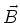with velocityexperiences a force,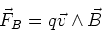(1)

Or equivalently,(2)

where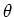is the angle between the velocity vector and the magnetic field vector. The direction of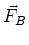is given by the right hand rule. Note the following:

(i) The force on the charged particle is always perpendicular to both the velocity vector and to the magnetic field vector.

(ii) If the particle moves in the direction of the magnetic field, it experiences no magnetic force.

(iii) If the particle moves perpendicular to the magnetic field it experiences the maximum force.

(iv) Since the force is perpendicular to the magnetic field lines and to the velocity vector, the particle spirals'' around the magnetic field lines. The larger the magnetic field the larger the magnetic force and the tighter the spiral.

(v) No work is done by the magnetic field on the charged particle. The kinetic energy of the particle is therefore a constant, if no other forces act on the charged particle.

Constantfield perpendicular toA useful notation for drawing vectors in three dimensions:

- A cross indicates a vector into the page

- A dot indicates a vector coming out of the page

Consider the simplest case in which a particle of charge q has velocitywhich is perpendicular to the direction of the magnetic field, then,

 FB = q v B (3)

The direction of this force is perpendicular to both the direction of the magnetic field and the direction of the velocity. It also has constant magnitude. The charged particle then undergoes circular motion, with,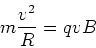(4)

This is an incredibly important phenomenon. A vast array of devices use the ability of magnetic fields to bend charges. Note however that a more precise theory finds that power is dissipated by an accelerating charge, including charges in uniform circular motion. This is why high energy particle accelerators have very large circumference. The radiation from an accelerated charged particle is proportional to the acceleration squared. An important use of this effect is for producing high intensity x-rays for a variety of purposes. Now look at various quantities that are important in the circular motion of a charge in a magnetic field:

(i) Radius of the orbit - R=mv/qB.

(ii) Period of the orbit -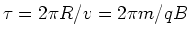.

(iii) Frequency (Cylotron frequency) of the orbit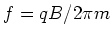.

Notice that the period and frequency of the orbit do not depend on the radius. They only depend on the charge to mass ratio q/m and the applied magnetic field B. In addition, if we know the speed of a charged particle and the magnetic field, we can find the charge to mass ratio. This is used in mass spectrometers. Often the frequency of this orbit is called the cyclotron frequency.

The circular motion described about is the basis of understanding more complex motion. For example

(i) If a charged particle has a component of its velocity parallel to the magnetic field lines, then it spirals around the magnetic field lines in a helical motion.

(ii) If there is a non-uniform magnetic field, then the radius of the spiral is larger in regions of weak field and smaller in regions of high field.

Electric and magnetic fields

If a charge moves through a region of space which has both electric and magnetic fields, then the particle experiences both the electric force (), as well as the magnetic force.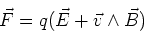(5)

which is the often called the Lorentz force.

A velocity selector

A charged particle in crossed electric and magnetic fields can still have constant velocity motion. This occurs if the electric and magnetic forces balance perfectly. This special case can be used as a velocity selector. That is, if we want to select particles with speed v from a set of particles with different speeds, we can do so by arranging crossed electric and magnetic fields in the correct manner. Here is how to do it: Choose,and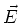all perpendicular to each other. The direction of the magnetic force is then either parallel or antiparallel with the electric force. If we choose the electric force to be antiparallel to the magnetic force, we can arrange for them to cancel each other. This is achieved if,

 qE = qvB (6)

Therefore particles with speed v will travel without deflection, provided,

 v = E/B (7)

Notice that this does not depend on the charge or the mass of the particles. However the selectivity does depend on the charge and the mass.

Thompson measurement of q/m of the electron

He used a velocity selector so that, v=E/B. In addition, he accelerated electrons in a cathode ray over a potential V, so that,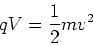(8)

which gives,(9)

solving for q/m yields,(10)

Since he knew E,V and B, he could find q/m. Later Millikan used his oil drop experiment to find e directly.